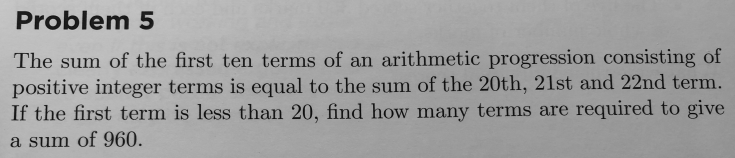# Arithmetic Sequence

#### Subhotosh Khan

##### Super Moderator
Staff member

View attachment 28205
What have you learned about arithmetic sequence?

If 'a' is the first number of the sequence and 'd' is the common difference -​
do you know the expression for common 'n' th element of the sequence?​
do you know the expression for the sum of the consecutive elements from term 'm' to 'n' (m>n)?​

#### Subhotosh Khan

##### Super Moderator
Staff member
I need help on the first question
What have you learned about arithmetic sequence?

#### JustYourAverageFriend

##### New member
What have you learned about arithmetic sequence?
Not much, but normally I can solve a question given the terms but this question doesn’t give terms in the sequence so I am not really sure the steps in solving this. Normally I would find the pattern and then apply it to any numbers I need to find but this isn’t the case.

#### topsquark

##### Senior Member
Not much, but normally I can solve a question given the terms but this question doesn’t give terms in the sequence so I am not really sure the steps in solving this. Normally I would find the pattern and then apply it to any numbers I need to find but this isn’t the case.
Start here:

$a_n = a_0 + nd$
What is $a_0 + a_1 + \text{ ... }$ in terms of $a_0$ and d?

What is $a_{20} + a_{21} + a_{22}$ in terms of $a_0$ and d?

-Dan

•HallsofIvy and JeffM

#### JustYourAverageFriend

##### New member
I’m sorry, but I don’t think I understand.

#### pka

##### Elite Member
In the first question an Arithmetic progression (AP) is [MATH]a_n=a_1+(k-1)d[/MATH] where [MATH]a_1[/MATH] is a positive integer and [MATH]d[/MATH] is the common difference. The sum of of the first [MATH]n[/MATH] terms is [MATH]\sum\limits_{k = 0}^{n - 1} {\left( {{a_1} + kd} \right)} = \frac{{n({a_1} + {a_n})}}{2}[/MATH]

•topsquark

#### JustYourAverageFriend

##### New member
I’m sorry, but I’m only an year 7 so I don’t really understand all these symbols. Sorry once again if it takes too much time to explain. Sorry

#### Subhotosh Khan

##### Super Moderator
Staff member
If 'a' is the first number of the sequence and 'd' is the common difference -do you know the expression for 'n' th element of the sequence?
Do you know the answer of the question posed above?

We are trying to figure out the first step of the explanation - so that it will make sense to you.

Last edited:

#### topsquark

##### Senior Member
I’m sorry, but I’m only an year 7 so I don’t really understand all these symbols. Sorry once again if it takes too much time to explain. Sorry
If you don't understand a the level of what they are asking then why have you been asked the question?

The sum of the first 10 terms in the sequence:
$(a_0) + (a_0 + d) + (a_0 + 2d) + \text{ ... } + (a_0 + 9d)$ is what? Write it out and combine like terms.

-Dan

#### Dr.Peterson

##### Elite Member
Which problem are you doing? I suppose you must mean #5,And what help do you need?

We need at least to know what tools you have available. What have you learned about arithmetic progressions, and possibly about number theory? What did you try, and what happened?

I would write an equation using whatever formulas you have learned for the sum of such a progression, which will have two variables. Solve as much as you can, and apply the results to the question asked. I haven't tried it yet, since I don't know what you are have available.

I’m sorry, but I’m only an year 7 so I don’t really understand all these symbols. Sorry once again if it takes too much time to explain. Sorry
So, what symbols do you understand? That's why you need to show us what you have learned.

Last edited:
•topsquark

#### JustYourAverageFriend

##### New member
If you don't understand a the level of what they are asking then why have you been asked the question?

The sum of the first 10 terms in the sequence:
$(a_0) + (a_0 + d) + (a_0 + 2d) + \text{ ... } + (a_0 + 9d)$ is what? Write it out and combine like terms.

-Dan
Would it be 10a0+ 45?

Last edited:

#### JustYourAverageFriend

##### New member
Do you know the answer of the question posed above?

We are trying to figure out the first step of the explanation - so that it will make sense to you.
Yes, I think so. To find the common difference generally you subtract two terms from each other.

#### Subhotosh Khan

##### Super Moderator
Staff member
Yes, I think so. To find the common difference generally you subtract two terms from each other.
I think you are misunderstanding the question.

#### JustYourAverageFriend

##### New member
Do you know the answer of the question posed above?

We are trying to figure out the first step of the explanation - so that it will make sense to you.
So what A would be?

#### JustYourAverageFriend

##### New member
If it’s how A would br in an equation would it be A1 (small one)

#### JustYourAverageFriend

##### New member
All good now, I figured it out thanks for your help!

#### Jomo

##### Elite Member
The first sentence gives you the following equation.

Let a = 1st term.
(a)+(a+d)+(a+2d)+ ... +(a+9d) = (a+19d) + (a+20d) + (a+21d)